# Quiz 2: Allied Office Products

Business

(a) Calculation of return on investment for Air-Seal product-line in 1985: Return on investment: Return on investment measures the percentage return on specified investment for measuring the profitability at a given point of time. Extracted information: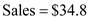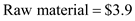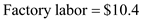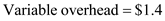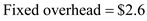Methodology: For calculating the return on investment, the formula is as follows: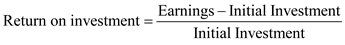Here,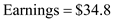and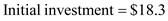. Substitute:Thus, it can be concluded that the return on investment for Air-Seal product line in 1985 is 0.009%. (b) Use DuPont formula to explain the performance of product-line: DuPont analysis explains that ROE (Return on equity) is affected by mainly three factors: • Operating efficiency: It is measured through profit margin (profit/sales) • Asset use efficiency: It is measured through total asset turnover (sales/assets) • Financial leverage: Lastly, it is measured through the equity multiplier (assets/equity). In the given case of AirWrap packaging equity variable data are not provided, so that a DuPont analysis of company's product line is not possible to do.

Excess capacity : A situation may be referred to excess capacity for a product line if actual production is lesser than what is optimal or achievable for that product line. This often means when demand is below then potentially supply of a particular product in the existing market. ROI : It is a performance measure used to calculate the efficiency of a particular investment or to number of investments. It is calculated as follows: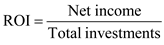Step 1: To calculate net profit for A product line in year 1985 If company working with its full capacity then income statement (Exhibit 1) is presented as follows for excess units' capacity: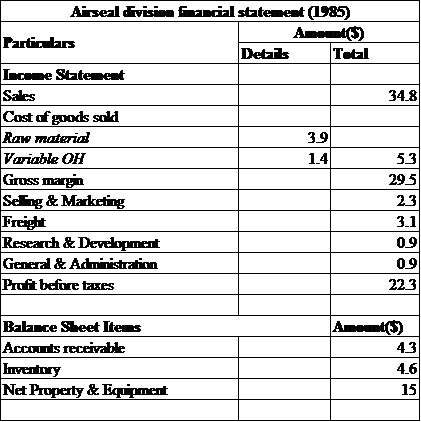Step 2: To calculate ROI;Substitute: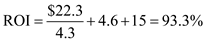Thus, the whole calculation estimates that if this company produced with its excess capacity fixed costs is not to be included in net income calculates. Therefore, due to this impact the amount of net income will increases and finally ROI will also increase.

(a) Calculation of break-even sales volume in dollars for Air-Seal product-line in 1985 : Break-even sales volume: Break-even sales volume refers to the amount of one's product that he/she will need to manufacture and sell for the purpose to cover its total production costs. Extracted information:Methodology: For calculating the break-even sales volume in dollars the formula is as follows: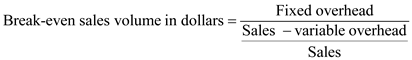Substitute:Thus, break-even sales volume in dollars for Air-Seal product-line in 1985 is \$2.71. (b) Operating Leverage: Operating leverage is used to measure the responsiveness of the profit as per the changes in sales volume. The higher operating leverage of a firm indicates that the firm's net income is more reactive to the changes in its sales volume. Usually, it is an indicator of a company's cost structure. There is a direct relationship between company's fixed costs and operating leverage. The higher the fixed costs (in relation to variable costs), the greater would be its operating leverage.